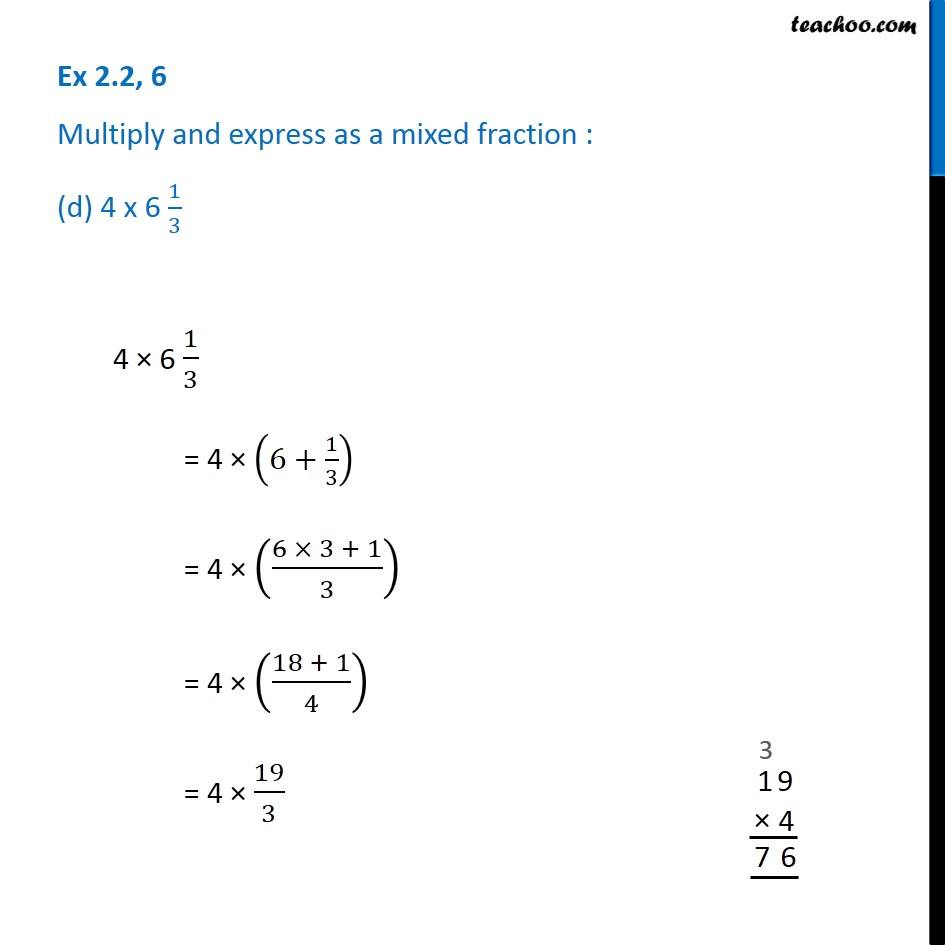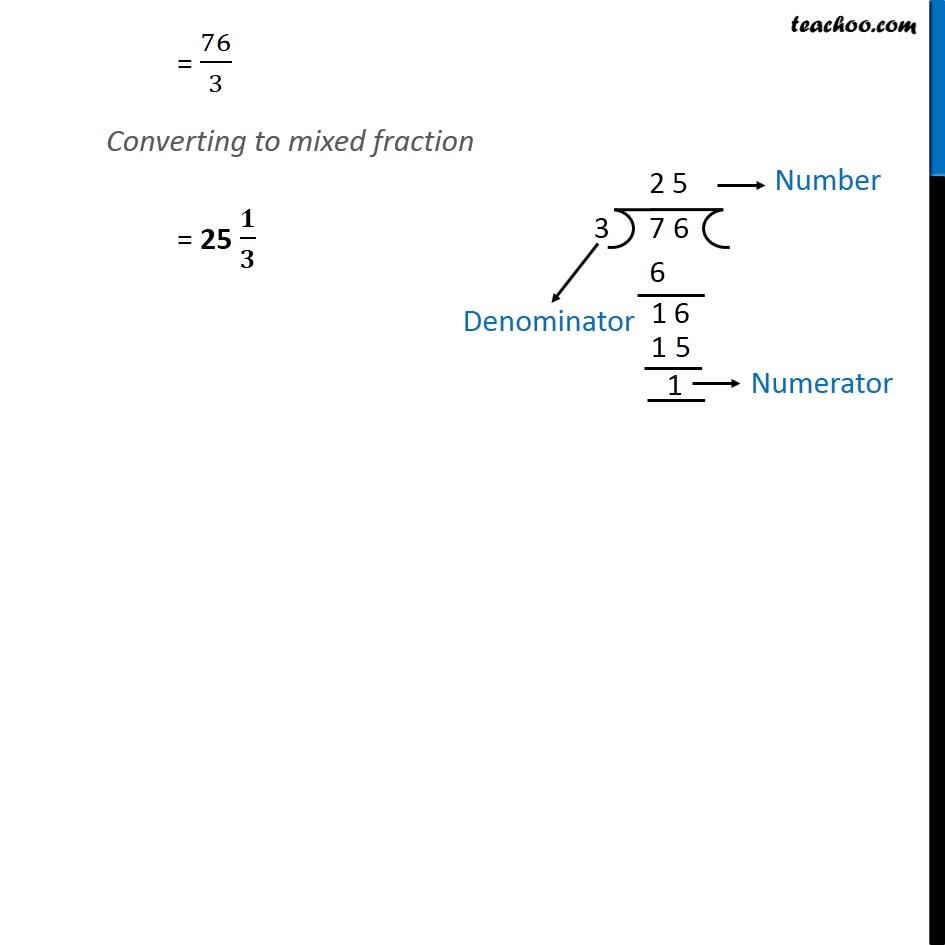Ex 2.2

Chapter 2 Class 7 Fractions and Decimals
Serial order wiseGet live Maths 1-on-1 Classs - Class 6 to 12

### Transcript

Ex 2.2, 6 Multiply and express as a mixed fraction : (d) 4 x 6 1/34 × 6 1/3 = 4 × (6+1/3) = 4 × ((6 × 3 + 1)/3) = 4 × ((18 + 1)/4) = 4 × 19/3 = 76/3 Converting to mixed fraction = 25 𝟏/𝟑 = 76/3 Converting to mixed fraction = 25 𝟏/𝟑